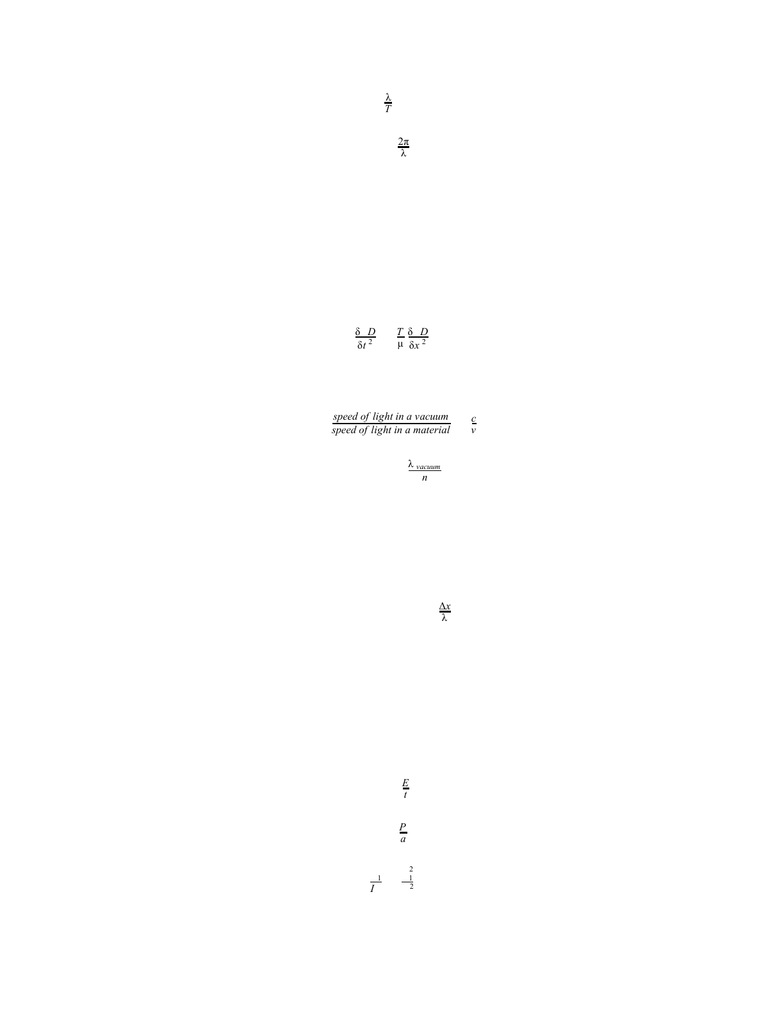Study Guides (380,000)
CA (150,000)
UTSC (10,000)
Final

# PHYA22H3 Study Guide - Final Guide: Electric Field, Eyepiece, Right-Hand RulePremium

Department
Physics and Astrophysics
Course Code
PHYA22H3
Professor
Daniel Weaver
Study Guide
Final

This preview shows pages 1-3. to view the full 28 pages of the document.University of Toronto
PHYA22
Physics II for the Life Sciences
Prof: Dan Weaver
Winter 2019
Final Exam
Exam Guide
Topics Included:
Waves
Superposition
Optics and Lenses
Electric Charges
Electric Fields
Electric Potential
Current and Circuits
Magnetism
Atomic Physics
Nuclear Physics
1

Only pages 1-3 are available for preview. Some parts have been intentionally blurred.

Subscribers OnlyChapter 16 - Travelling Waves
Types of Waves:
Transverse - displacement is perpendicular to the direction in which the wave travels
Longitudinal - particles in the medium are displaced parallel to the direction in which
the wave travels
Classes of Waves:
Mechanical - travel through a material
Electromagnetic - don’t require a material to travel and are able to travel through a
vacuum
Wave Speed: speed with which a wave travels down a medium
v=μ
T
where v = speed
T = tension
= linear densityμ
Linear Density: mass to length ratio of a string
μ = L
m
where = linear densityμ
m = mass
L = length
Snapshot Graph: wave’s displacement as a function of position at a a single time t
History Graph: wave’s displacement as a function of time at a single position x
Sinusoidal Waves: wave that oscillates with simple harmonic motion
Period (T) - time interval for one cycle of motion to occur
Amplitude (A) - maximum value of displacement
Wavelength ( ) - distance spanned by one cycle of motionλ
Wave number (k) - spatial frequency of wave
Angular frequency ( ) - angular displacement per unit timeω
T=f
1
2

Only pages 1-3 are available for preview. Some parts have been intentionally blurred.

Subscribers Onlyfv =λ
T= λ
k=λ
2π
k ω = v
(x, ) sin (kx t) D t =A− ω + ϕ
Wave Equation: Newton’s second law written for a continuous object where the displacement
is a function of position and time
δt 2
δ D
2=μ
T
δx 2
δ D
2
Index of Refraction: ratio between speed of light in a vacuum and speed of light in a material
n=speed of light in a vacuum
speed of light in a material =v
c
λ material =n
λ vacuum
Phase and Phase Difference:
Phase is constant throughout every point on a wave front
Depends on the ratio of the separation between two points on a wave and their
wavelength
ϕ kΔx π Δ = = 2 λ
Δx
Power (P): amount of energy transferred per unit time (t)
Intensity (I): power to area (a) ratio
Wave with a smaller area will have a larger intensity than another wave with equal
power that takes up a larger area
Is related to the radius (r)
Intensity is also proportional to the square of the amplitude of the wave
P=t
E
I=a
P
I 2
I 1=r 2
2
r 2
1
3